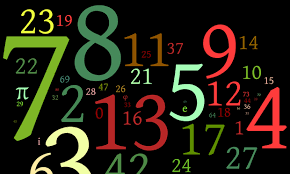# LSAT & Law School BlogThis week, we will focus on an example of how to setup an analytical reasoning (logic games) question. Our practice question will be from the June 2007 LSAT.

This is Section 1, Question 1:

A company employee generates a series of five-digit product codes in accordance with the following rules: The codes use the digits 0, 1, 2, 3, and 4, and no others. Each digit occurs exactly once in any code. The second digit has a value exactly twice that of the first digit. The value of the third digit is less than the value of the fifth digit.

Rule 1 - First, we will diagram this question by pulling out each rule we are given. The first one states that it is a five-digit code, so we will make 5 blank spaces.

__ __ __ __ __

Rule 2 - Next, we are told that these digits only use the digits 0-4 and no others.

0 1 2 3 4

Rule 3 - Then we are told each digit occurs only one time.

0 1 2 3 4

1 1 1 1 1

Rule 4 - The next rule is that the second digit has a value exactly twice that of the first digit, so:

x 2x _ _ _ : From this rule we can infer that there are only two possibilities once this rule is considered, either:

1 2 _ _ _ OR 2 4 _ _ _

Rule 5 - Our final rule is that the value of the third digit is less than the value of the fifth digit. From this we infer that the number “4” cannot be in space 3 and the number “0” cannot be in space 5.

Once you diagram it always is smart to make a few scenario possibilities with all the rules taken into account. So, we have:

#1: 1 2 0/3 _ 3/4 – This is compiling all the rules since we know the second number has to be twice the first, which works here with 1 & 2. It also takes into account that the third digit < fifth digit – and we only have the numbers 0, 3, and 4 left so this means that spots 3 and 5 will either be 0 & 3, 0 & 4, or 3 & 4.

#2: 2 4 0/1 _ 1/3 – Again, we are compiling all the rules since we know second number is twice the first, which works with 2 & 4. It also takes into account that third digit < fifth digit – and we only have the numbers 0, 1, and 3 left. So, this means that spots 3 and 5 will either be 0 & 1, 0 & 3, or 1 & 3.

With the rules down and the two scenarios already figured out, you will probably be able to already answer a question or two. Let’s try the first one together just to see how we will implement what we have diagrammed.

Question 1:

1. If the last digit of an acceptable product code is 1, it must be true that the

(A) first digit is 2

(B) second digit is 0

(C) third digit is 3

(D) fourth digit is 4

(E) fourth digit is 0

This is a “must be true” question and we must implement all of our previously stated rules in addition to the new one we are given, that the last digit has to be 1. Since we diagrammed and made example scenarios, this will be a piece of cake. We will use Scenario #2 here:

#2: 2 4 0/1 _ 1/3

Our new rule is that digit 5 must be 1, so:

2 4 0/1 _ 1

Since each digit can only be used once (Rule # 3), we know that the third digit will have to be 0, and the only digit left for digit 4 is a 3- so we have our final code:

2 4 0 3 1.

Now answering the question will be simple, we will just run through each answer choice and see which was holds to be true with the code we have created. Option (a) states that the first digit is 2, which is correct!

While you're working hard and studying practice questions, make sure you take some time to read up on current events in the law school world.